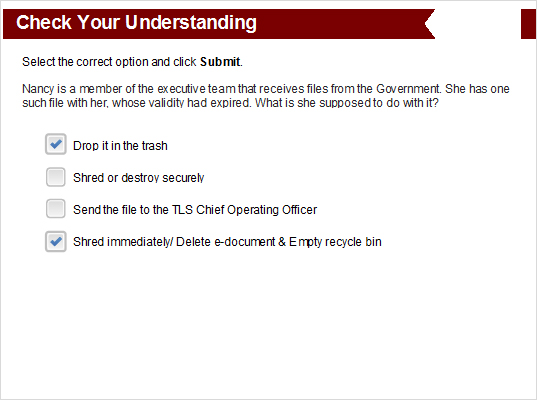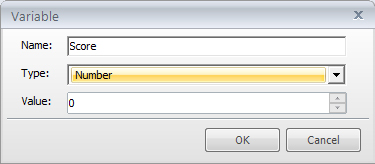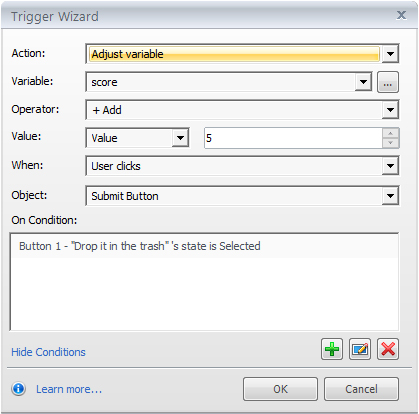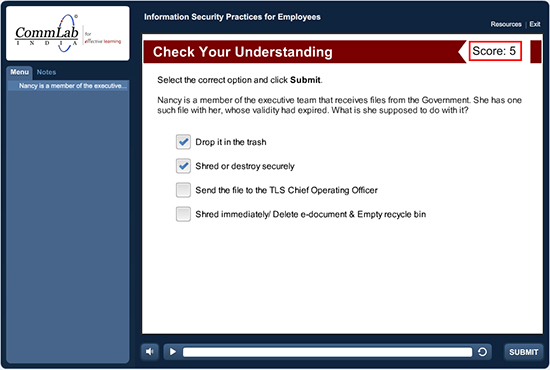# Articulate Storyline: Assigning Points to Each Correct Answer of a QuestionGenerally, in an e-learning course learners select all the correct answers then we assign the score for question. But we cannot assign the score for each correct answer.

One of our client’s requirement was to create a multiple response question and assign the score points for each correct answer. If the question have two correct answers when the learner select only one correct answer learner will get points.

We used the default features of Articulate Storyline to meet this requirement. Here are the steps.

Step 1:

Create a Multiple Response question as shown in the below screenshot.Note: Here, we have two correct answers i.e. Option1 and Option4.

Step 2:

Create a Numeric variable (Score) to calculate the score. Make sure that the initial value of the variable is 0. Refer to the screenshot below.Step 3:

Add a trigger to add 5 points to the variable Score when the learner selects a correct response and clicks the Submit button.Apply the same trigger to the other correct answer.

Step 4:

Publish the course and check the output.As you can see in the above screenshot, a score was assigned only to the correct option (Option1).

These are the steps to assign scores to one or some correct answers of an assessment question.

Hope you find this post useful. Do share your thoughts.